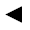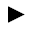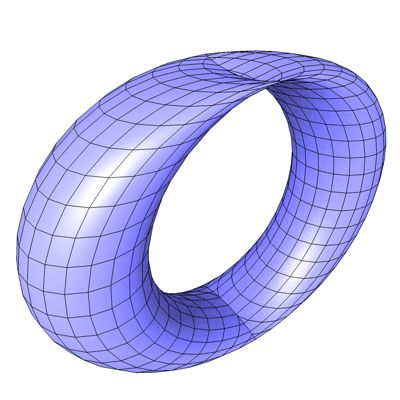[back]

## 3.1. Bohemian Dome

[next]The Bohemian Dome [ 1,7,11 ] is represented by the following equations.

 x = a cos(u) 3-7 y = b cos(v) + a sin(u) 3-8 z = csin(v) 3-9

The constants a, b and c determine the appearance of the figure.

To represent the area, the two parameters u and v must have the following values ​​(definition range).

 u is an element from the set of numbers [0, 2 pi] v is an element of the number set [0, 2 pi]

Since the Bohemian Dome is a closed figure, the definition range must be adhered to exactly, so it cannot be changed with the plugin.Fig. 5